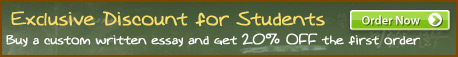### Solving Equations. Compares solving equations algebraically and by using matrices

Title: Solving Equations. Compares solving equations algebraically and by using matrices
Category: /Science & Technology/Mathematics
Details: Words: 417 | Pages: 2 (approximately 235 words/page)
Solving Equations. Compares solving equations algebraically and by using matrices
There are two main ways to solve equations, algebraically or by using matrices. There are also different subcategories of each way to solve the equations. Using matrices or solving algebraically has some differences and similarities. There are a few ways to solve equations algebraically. One line of attack is by substitution. In substitution, the goal is to solve for one of the variables in the first equation then substitute that variable into the second equation. …showed first 75 words of 417 total…
You are viewing only a small portion of the paper.